Next: Analysis in the time Up: MIDAS utilities for time Previous: Fourier analysis

Time series analysis in the frequency domain

For the detection of smooth signals, e.g. sinusoids, use either ORT/TSA with 'order'=1 or 2 harmonics, SCARGLE/TSA or AOV/TSA with 'order'=3 or 4 bins. The sensitivity of these statistics to sharp signals (such as strongly pulsed variations or light curves of very wide eclipsing binaries) is poor. For the detection of such signals better use ORT/TSA or AOV/TSA with the width of these features matched by the width of the of the top harmonics or the width of a phase bin, respectively.

The command SINEFIT/TSA serves two purposes: a) least squares estimation of the parameters of a detected signal and b) filtering the data for a given frequency (so-called prewhitening). The trend removal (zero frequency) constitutes a special case of this filtering. For a pure sinusoid model, thestatistic used in SINEFIT/TSA is related to that used in SCARGLE/TSA (Lomb, 1976, Scargle, 1982).

ORT/TSA -
Multiharmonic analysis of variance periodogram: The command computes the analysis of variance (AOV) periodogram for fitting data with a (multiharmonic) Fourier series. The fit of the Fourier series is done by a new efficient algorithm, employing projection onto orthogonal trigonometric polynomials. The results of the fit are evaluated using the AOV statistics, a powerful method newly adapted for the time series analysis (Schwarzenberg-Czerny, 1996, 1989). The model used in this method is the Fourier series of n harmonics. The resolution of the method may be tuned by change of n. Hence it is the method of choice for both smooth and sharp signals. The AOV statistic is the ratio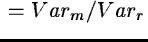. The distribution of S for white noise (Hohypothesis) and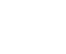order bins is the Fisher-Snedecor distribution F(2n+1,no-2n-1). The expected value of the AOV statistics for pure noise is 1 for uncorrelated observations and ncorr for observations correlated in groups of size ncorr.
SCARGLE/TSA -
Scargle sine model: This command computes Scargle's (1982) periodogram for unevenly spaced observations x. The Scargle statistic uses a pure sine model and is a special case of the power spectrum statistic normalized to the variance of the raw data,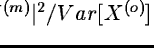. The phase origins of the sinusoids are for each frequency chosen in such a way that the sine and cosine components ofbecome independent. Hence for white noise (Ho hypothesis) S is the ratio of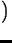and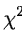. For large numbers of observations no, numerator and denominator become uncorrelated so that S has a Fisher-Snedecor distribution approaching an exponential distribution in the asymptotic limit: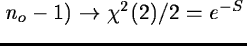for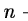.

We recommend this statistic for larger data sets and for the detection of smooth, nearly sinusoidal signals, since then its test power is large and the statistical properties are known. In particular the expected value is 1. For observations correlated in groups of size ncorr, divide the value of the Scargle statistics by ncorr(Sect. 12.2.4). The slow algorithm implemented here is suitable for modest numbers of observations. For a faster, FFT based version see Press and Rybicki (1991).

SINEFIT/TSA -
Least-squares sinewave fitting: This command fits sine (Fourier) series by nonlinear least squares iterations with simultaneous correction of the frequency. Its main applications are the evaluation of the significance of a detection, parameter estimation, and massaging of data. The values fitted for frequency and Fourier coefficients are displayed on the terminal. For observations correlated in groups of size ncorr multiply the errors by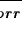(Sect. 12.2.4). With the latter correction and for purely sinusoidal variations SINEFIT/TSA computes the frequency with an accuracy comparable to the one of the power spectrum (Lomb, 1976, Schwarzenberg-Czerny, 1991). Additionally, the command displays the parameters of the fitted base sinusoid, i.e. of the first Fourier term.

SINEFIT/TSA returns also the table of the residuals X(r) (i.e. of the observations with the fitted oscillation subtracted) in a format suitable for further analysis by any method supported by the TSA package. In this way, the command can be used to perform a CLEAN-like analysis manually by removing individual oscillations one by one in the time domain (see Roberts et al., 1987, Gray & Desikhary, 1973). Since in most astronomical time series the number of different sinusoids present is quite small, we recommend this manual procedure rather than its automated implementation in frequency space by the CLEAN algorithm.

Alternatively, the command can be used to remove a trend from data. In order to use SINEFIT/TSA for a fixed frequency, specify one iteration only. The corresponding value ofmay in principle be recovered from the standard deviation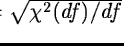, where df=nobs-nparm and nobs and nparm are the number of observations and the number of Fourier coefficients (including the mean value), respectively. However, the computation of the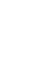periodogram with SINEFIT/TSA is very cumbersome while the results should correspond exactly to the Scargle periodogram (Scargle, 1982, Lomb, 1976).

AOV/TSA -
Analysis of variance for phase bins: The command computes the analysis of variance (AOV) periodogram for phase folded and binned data. The AOV statistics is a new and powerful method especially suitable for the detection of nonsinusoidal signals (Schwarzenberg-Czerny, 1989). It uses the step function model, i.e. phase binning. Its statistic is. The distribution of S for white noise (Hohypothesis) andorder bins is the Fisher-Snedecor distribution F(n-1,no-n), where n is number of bins. The expected value of the AOV statistics for pure noise is 1 for uncorrelated observations and ncorr for observations correlated in groups of size ncorr.

Among all statistics named in this chapter, AOV used by ORT/TSA and AOV/TSA is the only one with exactly known statistical properties even for small samples. On large samples, AOV is not less sensitive than other statistics using phase binning, i.e. the step function model:, Whittaker & Roberts and PDM. Therefore we recommend the ORT/TSA and AOV/TSA commands for samples of all sizes and particularly for signals with narrow sharp features (pulses, eclipses). If on the average ncorr consecutive observations are correlated, divide the value of the periodogram by ncorr and use the F(n-1,no/ncorr-n) distribution (Sect. 12.2.4). For smooth light curves use low order, e.g. 4 or 3, for optimal sensitivity. For numerous observations and sharp light curves use phase bins of width comparable to that of the narrow features (e.g. pulses, eclipses). Note that phase coverage and consequently quality of the statistics near 0 frequency are notoriously poor for most observations.Next: Analysis in the time Up: MIDAS utilities for time Previous: Fourier analysis
Petra Nass
1999-06-15